# expr command in Linux with examples

The expr command in Unix evaluates a given expression and displays its corresponding output. It is used for:

• Basic operations like addition, subtraction, multiplication, division, and modulus on integers.
• Evaluating regular expressions, string operations like substring, length of strings etc.

Syntax:

```\$expr expression
```

Options:

Below are some examples to demonstrate the use of “expr” command:

1. Using expr for basic arithmetic operations :

```\$expr 12 + 8
```

Example: Multiplication

```\$expr 12 \* 2
```

Output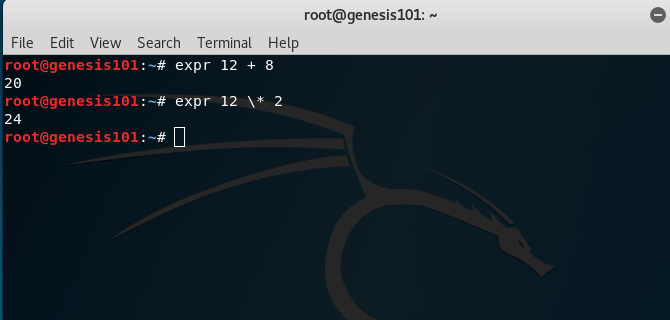Note:The multiplication operator * must be escaped when used in an arithmetic expression with expr.

2. Performing operations on variables inside a shell script

Example: Adding two numbers in a script

```echo "Enter two numbers"

sum=`expr \$x + \$y`

echo "Sum = \$sum"
```

Output: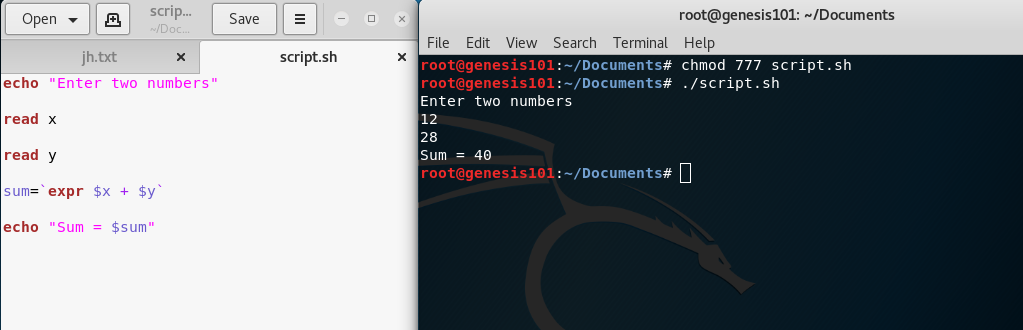Note: expr is an external program used by Bourne shell. It uses expr external program with the help of backtick. The backtick(`) is actually called command substitution.

3. Comparing two expressions

Example:

```x=10

y=20

# matching numbers with '='
res=`expr \$x = \$y`
echo \$res

# displays 1 when arg1 is less than arg2
res=`expr \$x \< \$y`
echo \$res

# display 1 when arg1 is not equal to arg2
res=`expr \$x \!= \$y`
echo \$res
```

Output: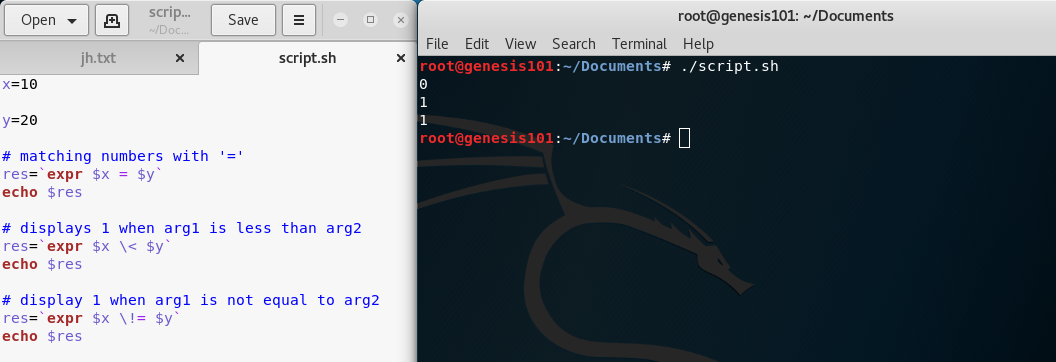Example: Evaluating boolean expressions

```# OR operation
\$expr length  "geekss"  "<"  5  "|"  19  -  6  ">"  10
```

Output: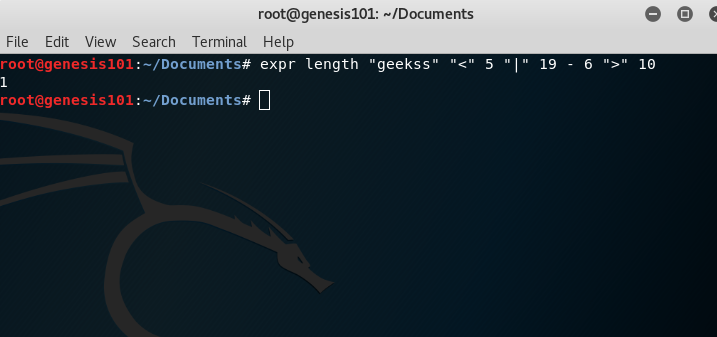```# AND operation
\$expr length  "geekss"  "<"  5  "&"  19  -  6  ">"  10
```

Output: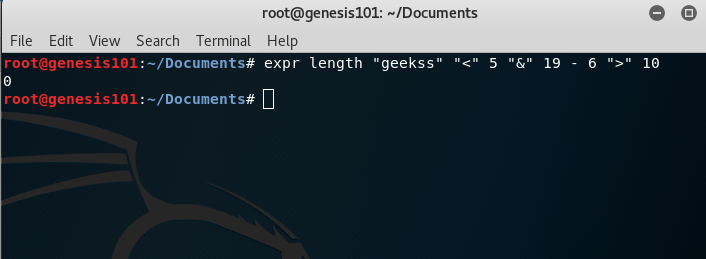4. For String operations

Example: Finding length of a string

```x=geeks

len=`expr length \$x`

echo \$len
```

Output: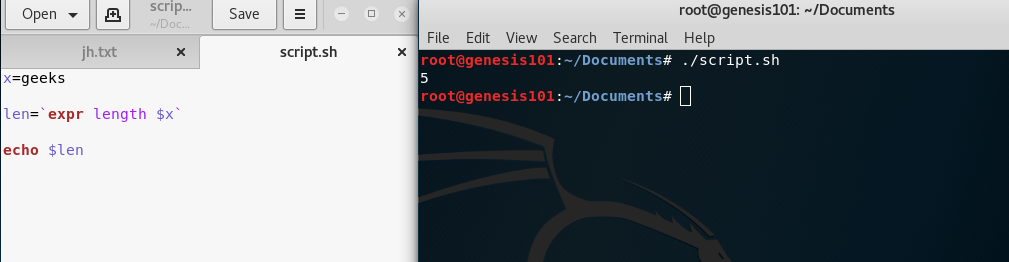Example: Finding substring of a string

```x=geeks

sub=`expr substr \$x 2 3`
#extract 3 characters starting from index 2

echo \$sub
```

Output: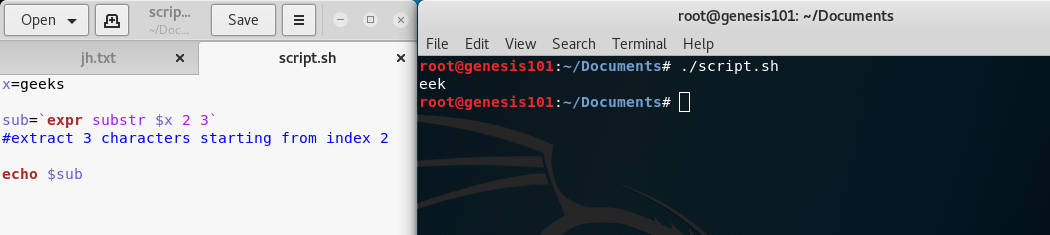Example: Matching number of characters in two strings

```\$ expr geeks : geek
```

Output: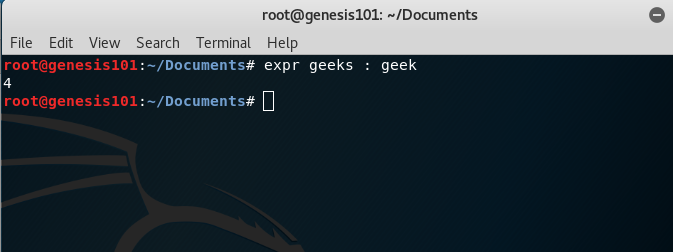My Personal Notes arrow_drop_upCheck out this Author's contributed articles.

If you like GeeksforGeeks and would like to contribute, you can also write an article using contribute.geeksforgeeks.org or mail your article to contribute@geeksforgeeks.org. See your article appearing on the GeeksforGeeks main page and help other Geeks.

Please Improve this article if you find anything incorrect by clicking on the "Improve Article" button below.

Article Tags :

Be the First to upvote.

Please write to us at contribute@geeksforgeeks.org to report any issue with the above content.